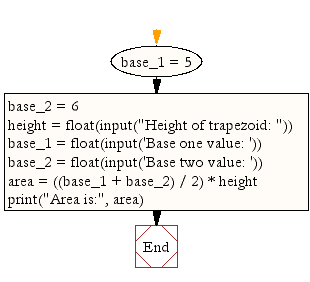﻿ Python Math: Calculate the area of a trapezoid - w3resource# Python Math: Calculate the area of a trapezoid

## Python Math: Exercise-3 with Solution

Write a Python program to calculate the area of a trapezoid.
Note: A trapezoid is a quadrilateral with two sides parallel. The trapezoid is equivalent to the British definition of the trapezium. An isosceles trapezoid is a trapezoid in which the base angles are equal so.

Sample Solution:-

Python Code:

``````base_1 = 5
base_2 = 6
height = float(input("Height of trapezoid: "))
base_1 = float(input('Base one value: '))
base_2 = float(input('Base two value: '))
area = ((base_1 + base_2) / 2) * height
print("Area is:", area)
```
```

Sample Output:

```Height of trapezoid: 6
Base one value: 10
Base two value: 5
Area is: 45.0
```

Pictorial Presentation:Flowchart:## Visualize Python code execution:

The following tool visualize what the computer is doing step-by-step as it executes the said program:

Python Code Editor:

Have another way to solve this solution? Contribute your code (and comments) through Disqus.

What is the difficulty level of this exercise?

Test your Python skills with w3resource's quiz

﻿

## Python: Tips of the Day

Python: Get the Key Whose Value Is Maximal in a Dictionary

```>>> model_scores = {'model_a': 100, 'model_z': 198, 'model_t': 150}
>>> # workaround
>>> keys, values = list(model_scores.keys()), list(model_scores.values())
>>> keys[values.index(max(values))]
'model_z'
>>> # one-line
>>> max(model_scores, key=model_scores.get)
'model_z'
```